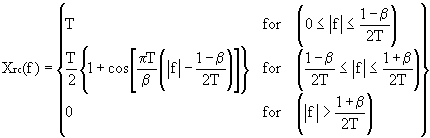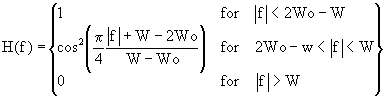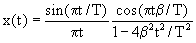### Raised-Cosine (RC) and Root-Raised-Cosine (RRC) filters are commonly used in digital data modems. Here, we provide design formulas for these filters.

#### Notes:

• Each version of the same formula is algebraically equivalent; they just use different parameters.
• The impulse response formulas include a “sinc(x)” term, that is, “sin(x)/x”. Although “sinc(0)” seemingly includes a divide-by-zero, L’Hospital’s rule indicates that the numeric value of sinc(0) is 1.0. Therefore, whenever you find a sinc(0) condition in using these formulas, just substitute 1.0.

### 1.1 Frequency Response

Version 1

Equation 9-2-26 of Digital Communications [Pro95]:Definitions:

• f is the frequency.
• T is the symbol time.
• beta is the rolloff factor.

Version 2

Equation 2.74 of Digital Communications: Fundamentals and Applications [Skl88]:Definitions:

• fis the frequency
• W – Wo is the excess bandwidth. (The rolloff factor is (W – Wo)/Wo.)

### 1.2 Impuse Response

Version 1

Equation 9-2-27 of Digital Communications [Pro95]:Definitions:

• t is the time.
• T is the symbol time.
• beta is the rolloff factor.

#### Version 2

The following appears in the book Digital Communications: Fundamentals and Applications [Skl88]:

```                       cos[2pi(W - Wo)t]
h(t) = 2Wo(sinc 2WoT) -------------------
1 - (4(W - Wo)t)^2```

( Note: The book is missing some parentheses in denomintor term which have been added here.)

Version 3

Contributed by Clay S. Turner

```        sin(bt)     cos(at)
h(t) =  ------- * ------------
bt      1-(2at/pi)^2

where b = 2 pi f
and   a = 2 pi excess bandwidth.
```

### 2.1 Frequency Response

Since the “root” aspect of a root-raised cosine filter is in the frequency domain, simply take the square root of the raised cosine frequency response given above to get the root-raised cosine frequency response.

### 2.2 Impulse Response

#### Version 1

Contributed by Clay S. Turner:

``````The RRC impulse response is given by:
h(t) =     pi^2      4at cos(t(a+b))+pi sin(t(b-a))
----------- * ------------------------------
pi(a-b)-4a         t(16t^2 a^2 - pi^2)
where b= 2 pi f   (f is usually half of your symbol rate)
and   a= 2 pi excess bandwidth
``````

#### Version 2

Contributed by Jim Shima:

``````total taps in filter are N (odd)

Ne = (N-1)/2

s = Ts/T
k = index

then,

for n=0.. N

let k = (n - Ne)  (make causal)

h(n) =  T * sin[  pi * (r-1) * k * s]
- 4 * r * Ts * k * cos[ pi*(r+1) * k*s]  }
--------------------------------------------------------------
T^(0.5) * pi * k * Ts * [ 16*r^2*k^2*s^2 - 1]

0/0 case:

h(n) = T^(-0.5)* [ 1 - r*(1 - (4/pi)) ]

must also take care of any x/0 cases.``````